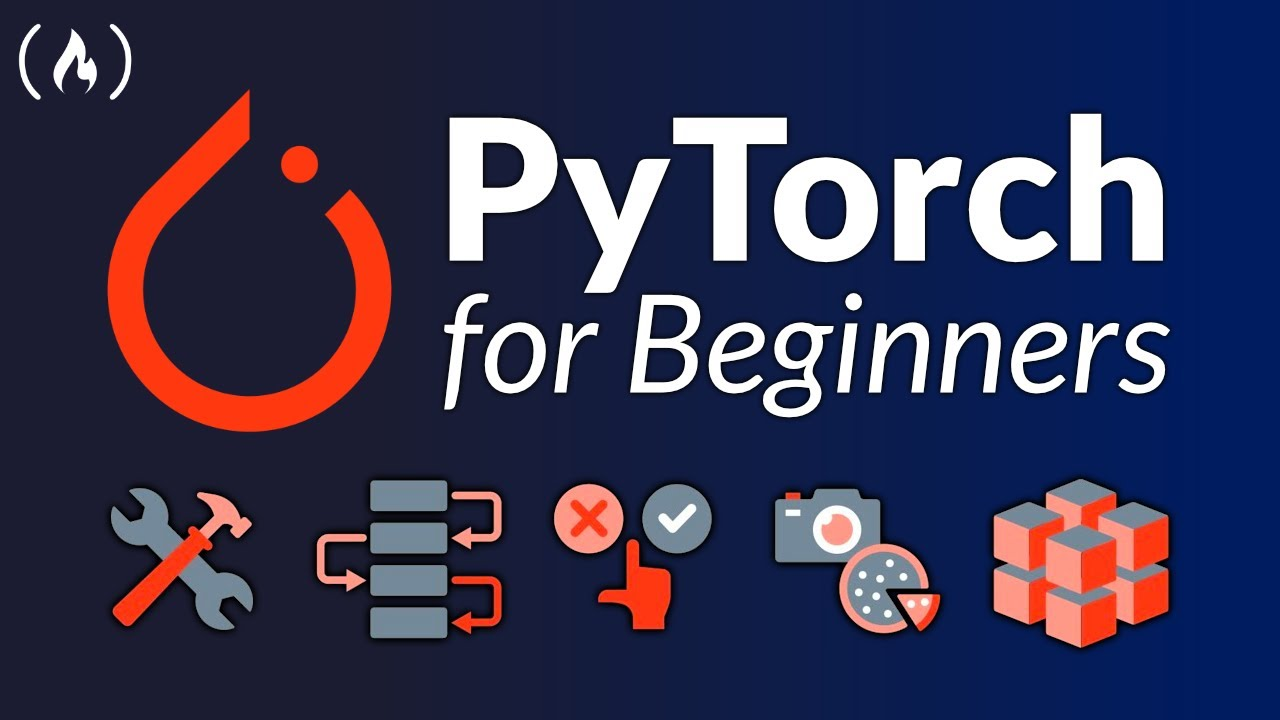# PyTorch for Deep Learning & Machine Learning – Full CourseLearn PyTorch for deep learning in this comprehensive course for beginners. PyTorch is a machine learning framework written in Python.

Learn PyTorch for deep learning in this comprehensive course for beginners. PyTorch is a machine learning framework written in Python.

Some sections below have been left out because of the YouTube limit for timestamps.

0:00:00 Introduction

🛠 Chapter 0 – PyTorch Fundamentals
0:01:45 0. Welcome and “what is deep learning?”
0:07:41 1. Why use machine/deep learning?
0:11:15 2. The number one rule of ML
0:16:55 3. Machine learning vs deep learning
0:23:02 4. Anatomy of neural networks
0:36:56 6. What can deep learning be used for?
0:43:18 7. What is/why PyTorch?
0:53:33 8. What are tensors?
0:57:52 9. Outline
1:03:56 10. How to (and how not to) approach this course
1:09:05 11. Important resources
1:14:28 12. Getting setup
1:22:08 13. Introduction to tensors
1:35:35 14. Creating tensors
1:54:01 17. Tensor datatypes
2:03:26 18. Tensor attributes (information about tensors)
2:11:50 19. Manipulating tensors
2:17:50 20. Matrix multiplication
2:48:18 23. Finding the min, max, mean & sum
2:57:48 25. Reshaping, viewing and stacking
3:11:31 26. Squeezing, unsqueezing and permuting
3:23:28 27. Selecting data (indexing)
3:33:01 28. PyTorch and NumPy
3:42:10 29. Reproducibility
3:52:58 30. Accessing a GPU
4:04:49 31. Setting up device agnostic code

🗺 Chapter 1 – PyTorch Workflow
4:17:27 33. Introduction to PyTorch Workflow
4:20:14 34. Getting setup
4:27:30 35. Creating a dataset with linear regression
4:37:12 36. Creating training and test sets (the most important concept in ML)
4:53:18 38. Creating our first PyTorch model
5:13:41 40. Discussing important model building classes
5:20:09 41. Checking out the internals of our model
5:30:01 42. Making predictions with our model
5:41:15 43. Training a model with PyTorch (intuition building)
5:49:31 44. Setting up a loss function and optimizer
6:02:24 45. PyTorch training loop intuition
6:40:05 48. Running our training loop epoch by epoch
6:49:31 49. Writing testing loop code
7:44:28 54. Putting everything together

🤨 Chapter 2 – Neural Network Classification
8:32:00 60. Introduction to machine learning classification
8:41:42 61. Classification input and outputs
8:50:50 62. Architecture of a classification neural network
9:09:41 64. Turing our data into tensors
9:25:58 66. Coding a neural network for classification data
9:43:55 68. Using torch.nn.Sequential
9:57:13 69. Loss, optimizer and evaluation functions for classification
10:12:05 70. From model logits to prediction probabilities to prediction labels
10:28:13 71. Train and test loops
10:57:55 73. Discussing options to improve a model
11:27:52 76. Creating a straight line dataset
11:46:02 78. Evaluating our model’s predictions
11:51:26 79. The missing piece – non-linearity
12:42:32 84. Putting it all together with a multiclass problem
13:24:09 88. Troubleshooting a mutli-class model

😎 Chapter 3 – Computer Vision
14:00:48 92. Introduction to computer vision
14:12:36 93. Computer vision input and outputs
14:22:46 94. What is a convolutional neural network?
14:27:49 95. TorchVision
14:37:10 96. Getting a computer vision dataset
15:01:34 98. Mini-batches
15:52:01 103. Training and testing loops for batched data
16:26:27 105. Running experiments on the GPU
16:30:14 106. Creating a model with non-linear functions
16:42:23 108. Creating a train/test loop
17:13:32 112. Convolutional neural networks (overview)
17:21:57 113. Coding a CNN
17:41:46 114. Breaking down nn.Conv2d/nn.MaxPool2d
18:29:02 118. Training our first CNN
18:44:22 120. Making predictions on random test samples
18:56:01 121. Plotting our best model predictions
19:19:34 123. Evaluating model predictions with a confusion matrix

🗃 Chapter 4 – Custom Datasets
19:44:05 126. Introduction to custom datasets
20:13:59 129. Becoming one with the data
20:39:11 132. Turning images into tensors
21:25:20 137. Creating a custom dataset class (overview)
21:42:29 139. Writing a custom dataset class from scratch
22:21:50 142. Turning custom datasets into DataLoaders
22:28:50 143. Data augmentation
22:43:14 144. Building a baseline model
23:11:07 147. Getting a summary of our model with torchinfo
23:17:46 148. Creating training and testing loop functions
23:50:59 151. Plotting model 0 loss curves
24:00:02 152. Overfitting and underfitting
24:32:31 155. Plotting model 1 loss curves
24:35:53 156. Plotting all the loss curves
24:46:50 157. Predicting on custom data